# NR Cell Performance with Downlink MU-MIMO

This example shows how to evaluate the system performance of a codebook-based downlink (DL) multi-user (MU) multiple-input multiple-output (MIMO) by using 5G Toolbox™ and the Communications Toolbox™ Wireless Network Simulation Library. The example uses link-to-system mapping-based abstract physical layer (PHY).

Using this example, you can

• Modify the MU-MIMO related scheduler configuration, and observe the impact on cell performance.

• Analyze cell performance for various channel delay profiles.

The simulation results show these key performance indicators (KPIs).

• Cell throughput

• Spectral efficiency

• Block error rate (BLER)

### Introduction

MU-MIMO is an important capability for increasing the capacity of a cellular network. Digital precoding facilitates MU-MIMO by spatially separating user transmissions over common time and frequency resources.

For DL MU-MIMO, a 5G NR base station (gNB) determines the precoder using one of these techniques:

• The gNB uses reported channel state information (CSI) Type II precoders.

• In a time division duplex (TDD) system, the gNB computes the DL precoder from the sounding reference signal (SRS) using channel reciprocity. For more information about this technique, see the TDD Reciprocity-Based PDSCH MU-MIMO Using SRS example.

In this example, the gNB facilitates DL MU-MIMO by using CSI Type II precoding.

This is the communication flow between the gNB and user equipment (UE) nodes.

• The gNB transmits the CSI reference signal (CSI-RS).

• UEs in the same cell estimate the channel from the CSI-RS.

• Each UE determines a rank indicator (RI), precoder matrix indicator (PMI), and channel quality indicator (CQI) from the estimated channel.

• UEs report RI, PMI, and CQI to the gNB.

• The CSI reported by the UE forms the basis for the downlink user-pairing algorithm.

• For the paired UEs, the gNB transmits precoded DL transmissions over common time and frequency resources.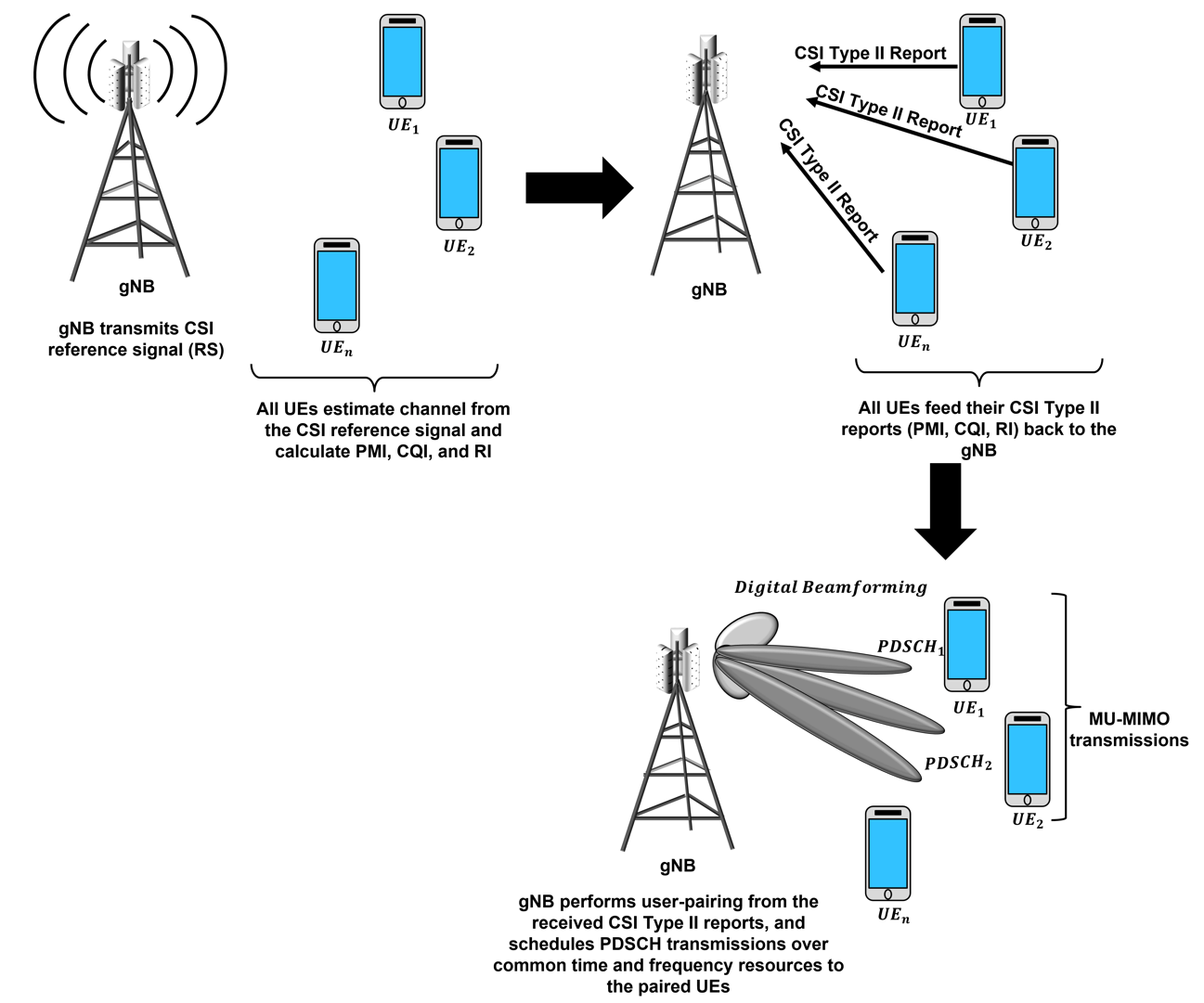### Simulation Assumptions

This example makes these assumptions.

• The number of CSI-RS ports equals the number of transmit antennas on the gNB.

• The round-robin scheduler pairs only UEs that report the same rank. Therefore, the scheduler does not pair two UEs if one of the UEs report a rank of `1` and the other UE reports a rank of `2`.

• The gNB transmits retransmission packets as a single-user (SU) MIMO.

• In this example, the scheduler does not support effective Modulation and Coding Scheme (MCS) calculation for paired users. The scheduler uses the MCS corresponding to the CQI reported by the UEs, which can result in higher BLER, especially when you set the `SemiOrthogonalityFactor` to a value less than `1`.

• The scheduler uses `SemiOrthogonalityFactor` and the reported PMI indices (i1) for user-pairing.

### Simulate Scenario

Check if the Communications Toolbox Wireless Network Simulation Library support package is installed. If the support package is not installed, MATLAB® returns an error with a link to download and install the support package.

`wirelessnetworkSupportPackageCheck`

Create a wireless network simulator.

```rng("default") % Reset the random number generator numFrameSimulation = 3; % Simulation time in terms of number of 10 ms frames networkSimulator = wirelessNetworkSimulator.init;```

Create the gNB. Specify the transmit power, subcarrier spacing, carrier frequency, channel bandwidth, number of transmit antennas, and receive gain of the gNB.

```gNBPosition = [0 0 0]; % [x y z] meters position in Cartesian coordinates gNB = nrGNB(Position=gNBPosition,TransmitPower=34,SubcarrierSpacing=15000, ... CarrierFrequency=2.6e9,ChannelBandwidth=5e6,NumTransmitAntennas=32,ReceiveGain=11); numUEs = 10; % Set the number of UEs```

Configure the DL MU-MIMO structure mu`MIMOConfigDL` by setting these parameters.

• `MinNumRBs` – Minimum number of resource blocks (RBs) that should be allocated to a UE to be considered for MU-MIMO.

• `MinCQI` – Minimum CQI for a UE to be considered as a MU-MIMO candidate. This examples uses the reported CQI indices, as defined in TS 38.214, Table 5.2.2.1-3 .

• `SemiOrthogonalityFactor` – Orthogonality between the reported PMI of the UEs, specified as a scalar in the range [0, 1]. `SemiOrthogonalityFacto`r value of `1` indicates that the scheduler pairs the users with only perfectly orthogonal precoders.

• `MaxNumUsersPaired` – Maximum number of users that can be paired for a MU-MIMO transmission.

```muMIMODLConfig = struct(MaxNumUsersPaired=4,MinNumRBs=2,SemiOrthogonalityFactor=0.9, ... MinCQI=10);```

Set these scheduler parameters by using the `configureScheduler` function.

• `ResourceAllocationType` – Physical downlink shared channel (PDSCH) resource allocation type, specified as `0` or `1`.

• `MaxNumUsersPerTTI` – Maximum number of users allocated per transmission time interval (TTI).

```configureScheduler(gNB,ResourceAllocationType=0,MaxNumUsersPerTTI=10, ... MUMIMOConfigDL=muMIMODLConfig);```

Calculate position of all UEs using these specifications.

• Relative distance of the UE from the gNB — `500` (Units are in meters)

• Sweep range of the azimuth angle in the horizontal plane — `[-60 60]` (Units are in degrees)

```% For all UEs, specify position in spherical coordinates (r,azimuth,elevation) % relative to gNB. ueRelPosition = [ones(numUEs,1)*500 (rand(numUEs,1)-0.5)*120 zeros(numUEs,1)]; % Convert spherical to Cartesian coordinates considering gNB position as origin [xPos,yPos,zPos] = sph2cart(deg2rad(ueRelPosition(:,2)),deg2rad(ueRelPosition(:,3)), ... ueRelPosition(:,1)); % Convert to absolute Cartesian coordinates uePositions = [xPos yPos zPos] + gNBPosition; ueNames = "UE-" + (1:size(uePositions,1));```

Create the UEs. Specify the name, position, receiver gain, and the number of receive antennas for each UE.

`UEs = nrUE(Name=ueNames,Position=uePositions,ReceiveGain=0,NumReceiveAntennas=1);`

Connect the UEs to the gNB, and configure full-buffer traffic in the DL direction.

`connectUE(gNB,UEs,FullBufferTraffic="DL")`

Add the gNB and UEs to the network simulator.

```addNodes(networkSimulator,gNB) addNodes(networkSimulator,UEs)```

Create an `N`-by-`N` matrix of link-level channels. `N` is the number of nodes in the cell. An element at index (i, j) contains the channel instance from node i to node j. An empty element at index (i, j) indicates that channel from node i to node j does not exist. i and j denote the node IDs.

```channelConfig = struct("DelayProfile","CDL-D","DelaySpread",100e-9); channels = createMultiUserCDLChannels(channelConfig,gNB,UEs);```

Create a custom channel model using `channels`. Install the custom channel on the simulator. The network simulator applies the channel for a packet in transit before passing the packet to the receiver.

```customChannelModel = hNRCustomChannelModel(channels); addChannelModel(networkSimulator,@customChannelModel.applyChannelModel)```

Set `enableTraces` to `true` to log the traces. If you set `enableTraces` to `false`, the simulator does not log traces. Setting `enableTraces` to `false` can speed up your simulation.

`enableTraces = true;`

Set up the scheduling logger and PHY logger.

```if enableTraces % Create an object for scheduler trace logging simSchedulingLogger = helperNRSchedulingLogger(numFrameSimulation,gNB,UEs); % Create an object for PHY trace logging simPhyLogger = helperNRPhyLogger(numFrameSimulation,gNB,UEs); end```

The example periodically updates the plotted metrics. Set the number of updates to perform during simulation.

```% This parameter has an impact on simulation time. numMetricsSteps = 10*numFrameSimulation;```

Set up a metric visualizer.

```metricsVisualizer = helperNRMetricsVisualizer(gNB,UEs,NumMetricsSteps=numMetricsSteps, ... PlotSchedulerMetrics=true,PlotPhyMetrics=true,PlotCDFMetrics=true,LinkDirection=0);```

Write the logs to MAT file. You can use these logs for post-simulation analysis.

`simulationLogFile = "simulationLogs"; % For logging the simulation traces`

Run the simulation for the specified number of frames `numFrameSimulation`.

```% Calculate the simulation duration (in seconds) simulationTime = numFrameSimulation*1e-2; % Run the simulation run(networkSimulator,simulationTime)```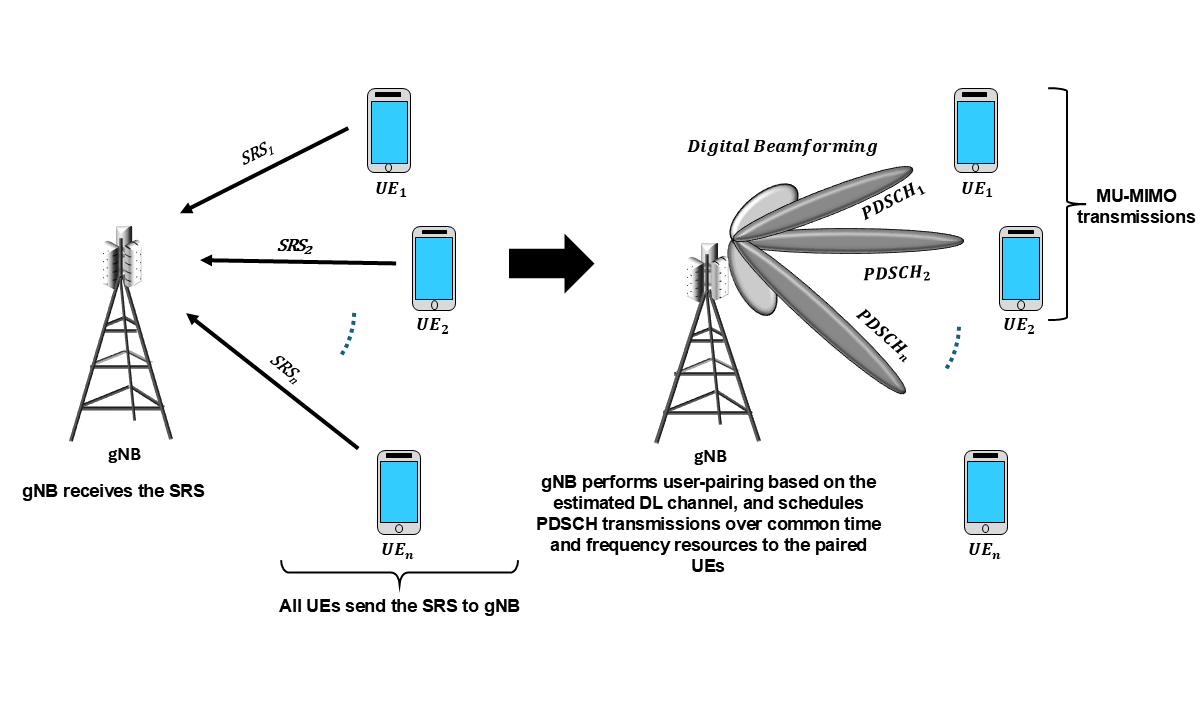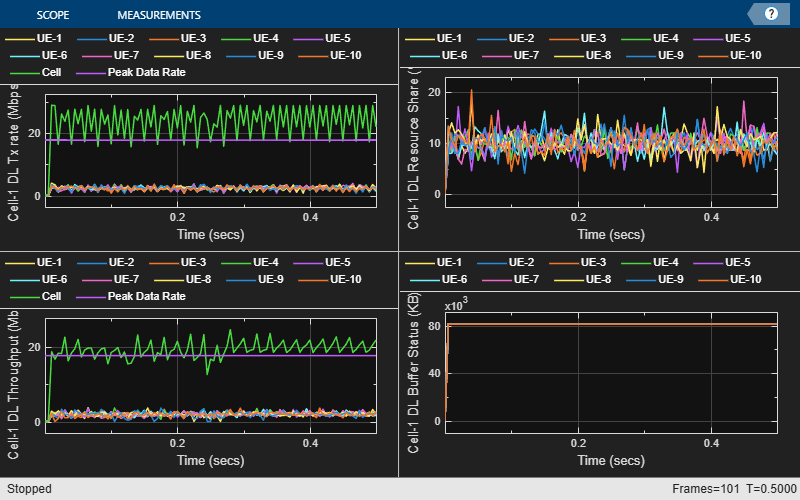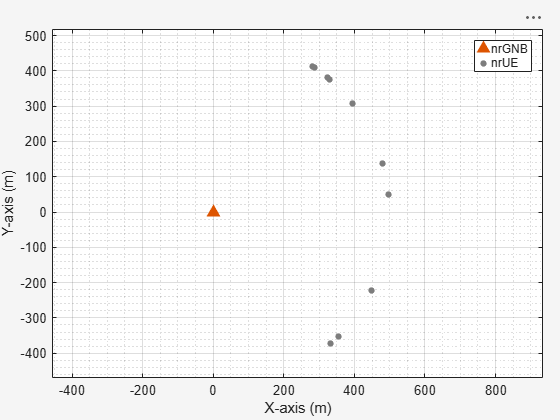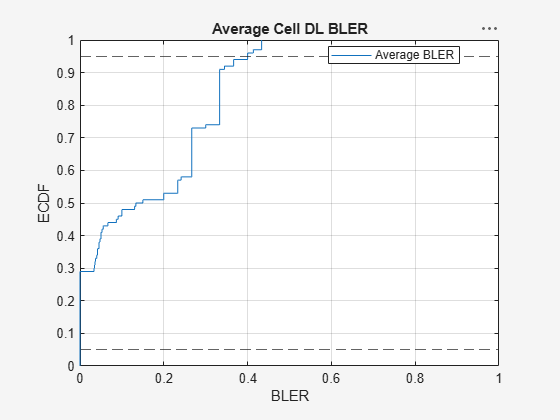### Results Summary

Display the system KPIs, including cell throughput, spectral efficiency and, empirical cumulative distribution function (ECDF) plots for cell throughput and average BLER. Using the ECDF plots, you can find the percentage of users with a throughput or BLER that is less than or equal to a value on the x-axis.

```% Read performance metrics displayPerformanceIndicators(metricsVisualizer)```
```Peak DL Throughput: 31.11 Mbps. Achieved Cell DL Throughput: 21.01 Mbps Achieved DL Throughput for each UE: [1.72 2.81 2 1.81 1.61 3.1 1.92 2.64 1.76 1.64] Peak DL spectral efficiency: 6.22 bits/s/Hz. Achieved DL spectral efficiency for cell: 4.20 bits/s/Hz Block error rate for each UE in the downlink direction: [0.04 0.074 0.074 0.222 0.077 0.483 0.483 0.222 0.08 0.08] ```
```if enableTraces simulationLogs = cell(1,1); if gNB.DuplexMode == "FDD" logInfo = struct("DLTimeStepLogs",[],"ULTimeStepLogs",[], ... "SchedulingAssignmentLogs",[],"PhyReceptionLogs",[]); [logInfo.DLTimeStepLogs,logInfo.ULTimeStepLogs] = getSchedulingLogs(simSchedulingLogger); else % TDD logInfo = struct("TimeStepLogs",[],"SchedulingAssignmentLogs",[],"PhyReceptionLogs",[]); logInfo.TimeStepLogs = getSchedulingLogs(simSchedulingLogger); end % Get the scheduling assignments log logInfo.SchedulingAssignmentLogs = getGrantLogs(simSchedulingLogger); % Get the PHY reception logs logInfo.PhyReceptionLogs = getReceptionLogs(simPhyLogger); % Save simulation logs in a MAT-file simulationLogs{1} = logInfo; save(simulationLogFile,"simulationLogs") end```

### Local Functions

Set up CDL channel instances for the cell.

```function channels = createMultiUserCDLChannels(channelConfig,gNB,UEs) numUEs = length(UEs); numNodes = length(gNB) + numUEs; channels = cell(numNodes,numNodes); waveformInfo = nrOFDMInfo(gNB.NumResourceBlocks,gNB.SubcarrierSpacing/1e3); sampleRate = waveformInfo.SampleRate; % Create a CDL channel model object configured with the desired delay % profile, delay spread, and Doppler frequency channel = nrCDLChannel; channel.CarrierFrequency = gNB.CarrierFrequency; % Delay profile. You can configure it as CDL- A,B,C,D,E channel.DelayProfile = channelConfig.DelayProfile; channel.DelaySpread = channelConfig.DelaySpread; % Delay Spread % Configure antenna down-tilt as 12 (degrees) channel.TransmitArrayOrientation = [0 12 0]'; channel.SampleRate = sampleRate; channel.ChannelFiltering = false; % For each UE set DL channel instance for ueIdx = 1:numUEs % Create a copy of the original channel cdl = hMakeCustomCDL(channel); % Configure the channel seed based on the UE number % (results in independent fading for each UE) cdl.Seed = 73 + (ueIdx - 1); % Set antenna panel cdl = hArrayGeometry(cdl,gNB.NumTransmitAntennas,UEs(ueIdx). ... NumReceiveAntennas,"downlink"); % Compute the LOS angle from gNB to UE [~,depAngle] = rangeangle(UEs(ueIdx).Position', ... gNB.Position'); % Configure the azimuth and zenith angle offsets for this UE cdl.AnglesAoD(:) = cdl.AnglesAoD(:) + depAngle(1); % Convert elevation angle to zenith angle cdl.AnglesZoD(:) = cdl.AnglesZoD(:) - cdl.AnglesZoD(1) + (90 - depAngle(2)); channels{gNB.ID,UEs(ueIdx).ID} = cdl; end end```

### Further Explorations

Try running the example for these use cases.

1. Analyze the impact of various CDL delay profiles (CDL-A/B/C/D/E) on the user-pairing strategy and system KPIs.

2. Analyze the impact of antenna configurations, such as CSI antenna ports, panel configuration, antenna orientation, and channel delay profile, on the system KPIs.

3. Modify the DL MU-MIMO configuration, and observe the impact on the system KPIs.

This list shows the relationship between `SemiOrthogonalityFactor` and BLER for a static scenario with 40 UEs. This simulation setup does not compute effective MCS if the scheduler pairs the users.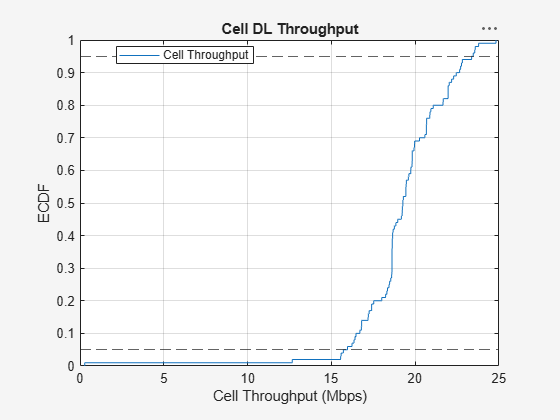This figure illustrates the impact of the `SemiOrthogonalityFactor` on cell throughput and average BLER. For the considered scenario, orthogonal users do not exist when you set `SemiOrthogonalityFactor` to `1` and use CSI Type II precoder.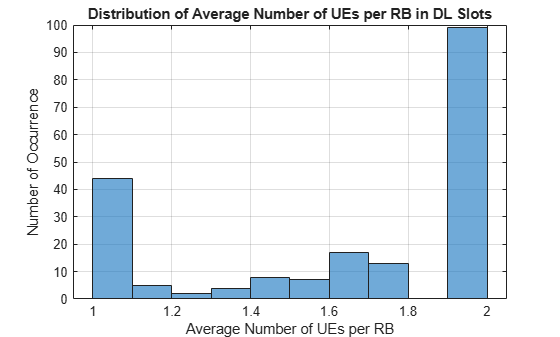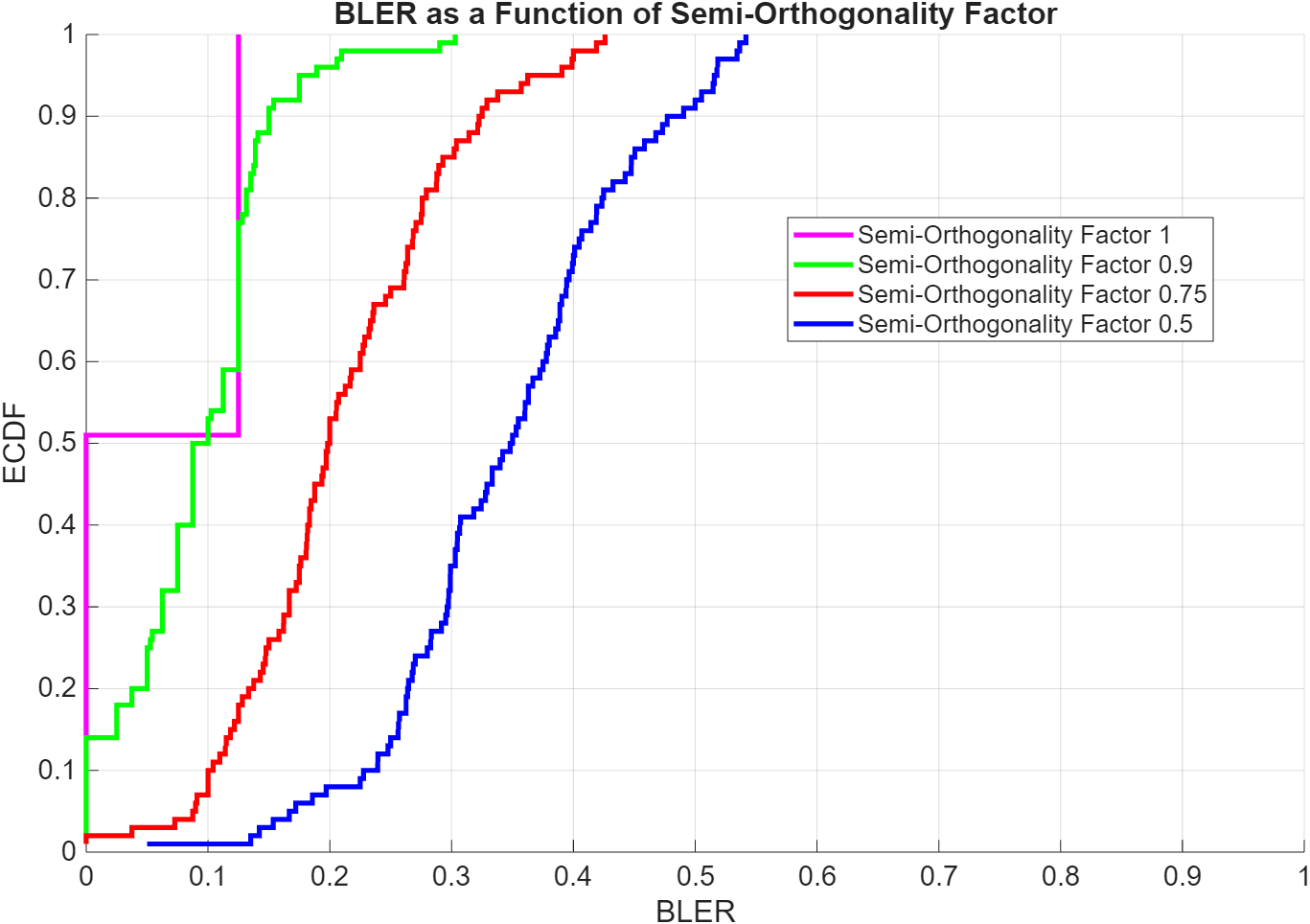### Appendix

The example uses these helper classes and functions:

### References

1. 3GPP TS 38.214. "NR; Physical layer procedures for data." 3rd Generation Partnership Project; Technical Specification Group Radio Access Network.